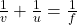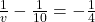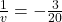Question

If an object is placed at a distance of 10 cm in front of a concave mirror of focal length 4 cm, find the position and characteristics of the image formed. Also, find the magnification.

1.Explanation:

Focal length f = – 4 cm

Object distance u = – 10 cm

v , image distance = ?

Mirror formulaPutting the given valuesv = – 6.67 cm .

magnification

m = v / u

= – 6.67 / – 10

= .667

so image will be smaller in size in comparison with size of object .

Characteristics will be that ,

1 ) it will be inverted and

2 ) it will be real image .